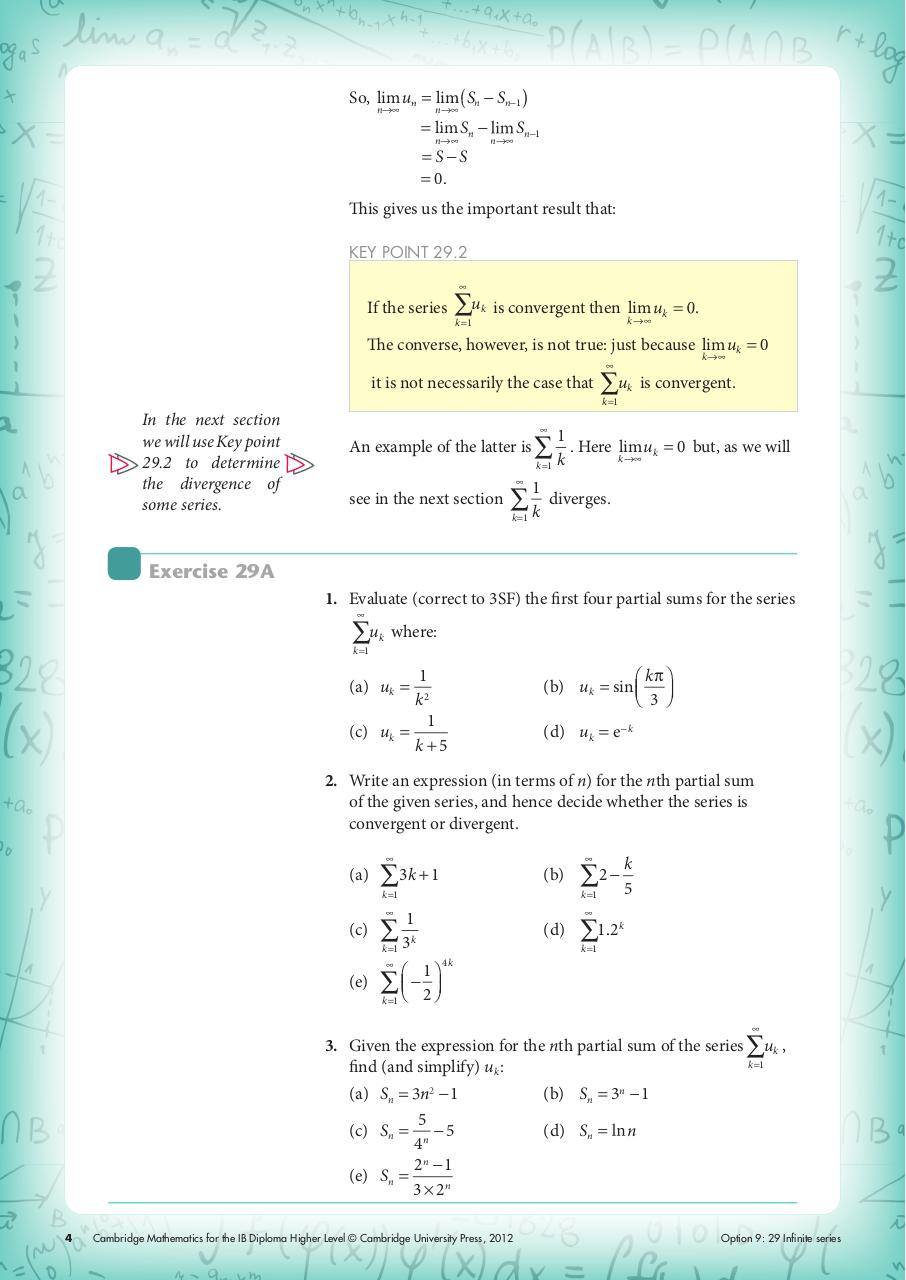# ch12.pdfPage 12326

#### Text preview

So, lim un
n→∞

lim
l ( Sn − Sn−1 )

n→∞

= lim Sn − l
n→∞

n→∞

= S−S
= 0.

Sn−1

This gives us the important result that:
KEY POINT 29.2

If the series

∑u
k =1

k

is convergent then lim

k →∞

k

0.

The converse, however, is not true: just because lim uk = 0
k →∞

it is not necessarily the case that

∑u

is convergent.

k

k =1

In the next section
we will use Key point
29.2 to determine
the divergence of
some series.

1
An example of the latter is ∑ . Here lim uk = 0 but, as we will
k →∞
k =1 k

see in the next section

1

∑k

diverges.

k =1

Exercise 29A
1. Evaluate (correct to 3SF) the first four partial sums for the series

∑u

k

where:

k =1

1
k2
1
(c) uk =
k+5
(a) uk =

(b) uk = sin

⎛ kπ ⎞
⎝ 3⎠

(d) uk = e − k

2. Write an expression (in terms of n) for the nth partial sum
of the given series, and hence decide whether the series is
convergent or divergent.

(a)

∑3k + 1

(b)

k =1

k =1

(c)
(e)

1
∑ 3k
k =1

⎛ 1⎞

∑ ⎝ − 2⎠

k

∑2 − 5

(d)

∑1.2

k

k =1

4k

k =1

3. Given the expression for the nth partial sum of the series ∑uk ,
k =1
find (and simplify) uk :
n2 − 1
5
(c) Sn = n − 5
4
2n − 1
(e) Sn =
3 × 2n
(a) Sn

4

Cambridge Mathematics for the IB Diploma Higher Level © Cambridge University Press, 2012

(b) Sn = 3n − 1
(d) Sn

l n

Option 9: 29 Infinite series